Expression of a variable from formula - math word problems - page 16

1. A mast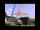A mast 32 meters high was broken by the wind so that its top touches the ground 16 meters from the pole. The still standing part of the mast, the broken part and the ground form a rectangular triangle. At what height was the mast broken?
2. RectangleCalculate area of the rectangle if its length is 12 cm longer than its width and length is equal to the square of its width.
3. Cargo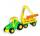Car with cargo weight 7610 kg. Weight of car is 23% of the total weight of car and cargo. How heavy is cargo?
4. Rectangular triangle PQRIn the rectangular triangle PQR, the PQ leg is divided by the X point into two segments of which longer is 25cm long. The second leg PR has a length 16 cm. The length of the RX is 20 cm. Calculate the length p of side RQ. The result is round to 2 decimal
5. Diagonal of squareCalculate the side of a square when its diagonal is 10 cm.
6. Determine AP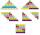Determine the difference of the arithmetic progression if a3 = 7, and a4 + a5 = 71
7. Geometric seqFind the third member of geometric progression if a1 + a2 = 36 and a1 + a3 = 90. Calculate its quotient.
8. DiagonalsGiven a rhombus ABCD with a diagonalsl length of 8 cm and 12 cm. Calculate the side length and content of the rhombus.
9. Red ballsIn the bag there are 3 red, 12 blue and 8 green balls. How many red balls we must be attached to the bag if we want the probability of pulling out the red balls was 20%?
10. Two machinesPerformances of two machines are in a ratio of 7:12. A machine with less power produced 406 pieces of products per shift. a) How many pieces produced per shift second machine? b) How many pieces produced two machines together for five shifts?
11. Quotient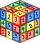Determine the quotient and the second member of the geometric progression where a3=10, a1+a2=-1,6 a1-a2=2,4.
12. Equilateral triangle ABCIn the equilateral triangle ABC, K is the center of the AB side, the L point lies on one-third of the BC side near the point C, and the point M lies in the one-third of the side of the AC side closer to the point A. Find what part of the ABC triangle conta
13. Coach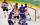Average age of 24 players and a coach of one team is 24 years. The average age of players without a coach is 23 years. How old is the coach?
14. HotelThe hotel has a p floors each floor has i rooms from which the third are single and the others are double. Represents the number of beds in hotel.
15. ChordIt is given to a circle k(r=6 cm) and the points A, B such that / AB / = 8 cm lies on k. Calculate the distance of the center of circle S to the midpoint C of the segment AB.
16. The gardenerThe gardener bought trees for 960 CZK. If every tree were cheaper by 12 CZK, he would have gotten four more trees for the same money. How many trees did he buy?
17. Q of GP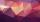Calculate quotient of geometric progression if a1=5 a a1+a2=12.
18. Ratio iso triangleThe ratio of the sides of an isosceles triangle is 7:6:7 Find the base angle to the nearest answer correct to 3 significant figure.
19. ParallelogramCalculate the missing side of a parallelogram, if you know the perimeter o and one side: a) o = 7.2 cm; b = 1.8 cm b) o = 5.4 cm; a = 1.9 cm
20. Right triangle eq2Find the lengths of the sides and the angles in the right triangle. Given area S = 210 and perimeter o = 70.

Do you have an interesting mathematical word problem that you can't solve it? Enter it, and we can try to solve it.

To this e-mail address, we will reply solution; solved examples are also published here. Please enter e-mail correctly and check whether you don't have a full mailbox.